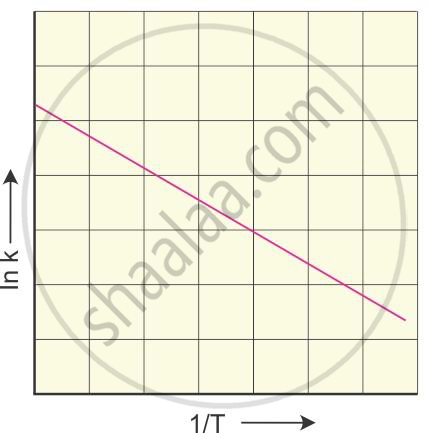# Explain a graphical method to determine activation energy of a reaction - Chemistry

Explain a graphical method to determine activation energy of a reaction.

#### Solution

The Arrhenius equation is given by

k=Ae^((-E_a)/(RT))

On taking logarithms of both sides, we get

log_nk=log_nA-E_a/(RT)

or

log_10k=-E_a/(2.303RT)+log_10A

log_10k=-E_a/(2.303R) times (1/T)+log_10A

The rate constant of a reaction is determined at various temperatures.

log10 k is plotted against the reciprocal of temperature. The graphical
representation isThe slope of the straight line graph is

–(Ea)/(2.303R)

from which the activation energy can be calculated.

Concept: Temperature Dependence of the Rate of a Reaction
Is there an error in this question or solution?